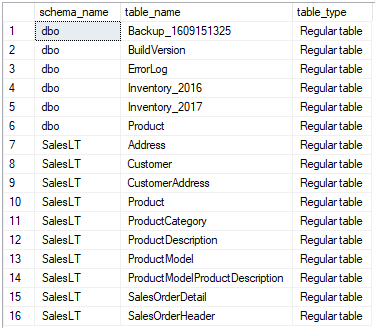## Identify table types in Azure SQL Database

Article for:

The query below lists tables in the current database and identifies its type. Types include:

• Plan old Regular table
• Graph node table
• Graph edge table
• System versioned table (temporal table)
• History table
• External table
• File table

## Query

``````select schema_name(schema_id) as schema_name,
name as table_name,
case when is_external = 1 then 'External table'
when is_node = 1 then 'Graph node table'
when is_edge = 1 then 'Graph edge table'
when temporal_type = 2 then 'System versioned table'
when temporal_type = 1 then 'History table'
when is_filetable = 1 then 'File table'
else 'Regular table'
end as table_type
from sys.tables
order by schema_name, table_name
``````

## Columns

• schema_name - name of the schema
• table_name - name of the table
• table_type - type of table identified by the query. Values include:
• Regular table
• Graph node table
• Graph edge table
• System versioned table
• History table
• External table
• File table

## Rows

• One row: represents one table in a database
• Scope of rows: all tables in a database
• Ordered by: schema and table name

## Sample results